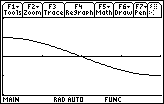Module 17 - Applications of Integration

Introduction | Lesson 1 | Lesson 2 | Lesson 3 | Self-Test

Lesson 17.1: Net Area

In previous modules you used the definite integral to find the area bounded by a function and the x-axis. In each case the graph of the function was above the x-axis. In this lesson you will see what happens when the function dips below the x-axis. You will also investigate the concept of the definite integral as a net area function.

All the curves explored in Module 16 were above the x-axis and the net area functions were always positive and increasing. The following investigates a definite integral when part of the curve is below the x-axis.

• Graph y = sin x in a [0, 2] x [-1, 1] window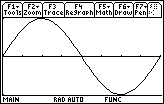• Evaluate the integral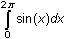by using the integral key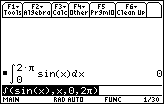How can the result be zero? The area bounded by y = sin x and the x-axis certainly is not zero. To help answer this question, break the interval of integration into two subintervals that represent the areas above and below the x-axis: (0,) and (, 2).

• Evaluate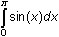and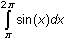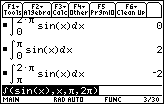Finding Positive and Negative Integrals

Review the graph of y = sin x and the values of the definite integralsand.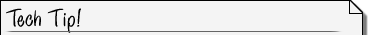Toggle between Screens You can toggle between the Home screen and the Graph screen by pressing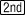.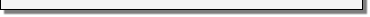The graph from 0 tois above the x-axis and the corresponding definite integral is positive.

The graph fromto 2is below the x-axis and the corresponding definite integral is negative.

Finding Net Area

The definite integralrepresents the value of the net area, or the area above the x-axis minus the area below the x-axis. The net area between the curve y = sin x and the x-axis is zero because it is the sum of 2, the area above the axis, and –2, the negative of the area below the axis.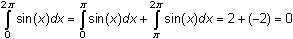17.1.1 Use the definite integral feature in the F5:Math menu of the Graph screen to approximate the values of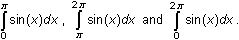Visualizing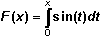You may obtain the general shape of a corresponding net area function on the interval [0, 2] by examining the graph of y = sin x.

• Refresh the graph of y = sin x by pressing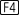The following characteristics of the net area function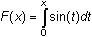can be determined from the graph of the curve y = sin x. Look at the graph while reading the tables below.

 Interval Curve Function Area Function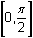and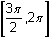increasing concave upward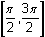decreasing concave downward

Using the fact that
the value of the curve function is the derivative of the net area function,
the x-values of maximums, minimums and points of inflection can be identified by examining how the curve is changing.

 x-value Curve Function is Changing Area Function x =from positive to negative maximum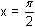from increasing to decreasing point of inflection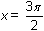from decreasing to increasing point of inflection

Other characteristics of the net area function include:

1. The net area when x = 0 is 0.
2. The net area on the interval [0,] is 2 and the net area function begins to decrease at that point, so the maximum of the net area function is 2 when x =.
3. The net area on the interval [0, 2] is 0, so the net area function is 0 when x = 2.

With these characteristics you can draw a graph of the net area function, as shown below together with the graph of the curve.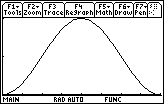y = sin xVisualizing the general shape of the integral function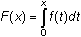is often very helpful.

Extending the Procedure to Other Curves

17.1.2 Graph the curve and find the net area bounded by y = x3 – 3x2x + 3 and the x-axis on the interval [0, 4].

Visualizing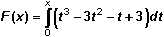The characteristics of the net area function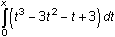can be found by examining the graph of the curve function. The graph of the curve is shown below in a [0, 4] x [ -5, 15] window with a list of the net area function's characteristics.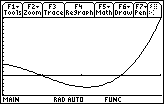y = x3 – 3x2 – x + 3 When x = 0 the net area function is 0. The net area function is concave downward on (0, 2.1547) because the curve is decreasing there. The net area function is concave upward on (2.1547, 4) because the curve is increasing there. Because the curve changes from positive to negative at x = 1, the net area function has a local maximum there. Because the curve changes from negative to positive at x = 3, the net area function has a local minimum there. The area from 0 to 1 appears to be the same as the area from 1 to 2, so the net area function is 0 when x = 2. This can be confirmed by evaluating the integral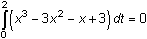From 2 to somewhere beyond 3 the net area function is negative because more of the area is below the x-axis to that point. Somewhere beyond 3 the net area function becomes positive because more area is above the x-axis than below it.

The graphs of the curve function and the net area function are shown below for comparison.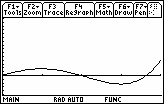y = x3 – 3x2 – x + 3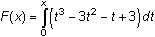17.1.3 Approximate the graph of a net area function that corresponds to the following curve from 0 to 4.